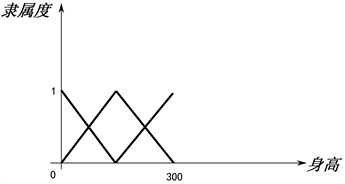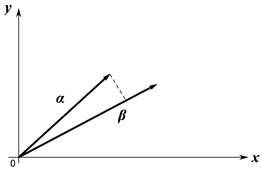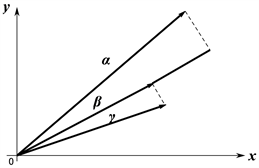﻿ 基于公理化模糊集合的投影相似度多属性决策方法

基于公理化模糊集合的投影相似度多属性决策方法Projection Similarity Multi-Attribute Decision-Making Method Based on Axiomatic Fuzzy Sets

Abstract: Aiming at the problem of fuzzy multi-attribute decision-making in which the attribute value is a linguistic value, this paper proposes a projection similarity multi-attribute decision-making method based on axiomatic fuzzy sets, which transforms the evaluation natural language into corresponding axiomization generated by fuzzy division Fuzzy set: Regarding the known alternatives as a vector, based on the modulus length and cosine value of the axiomatic fuzzy set vector, the projection similarity is proposed; and the corresponding multi-attribute decision-making model is constructed from this. Finally, the effectiveness and practicability of the model are illustrated by the comparison of calculation examples.

1. 引言

2. 公理化模糊集合的基本理论

$\stackrel{˜}{U}=\left\{{\mu }_{{A}_{1}}\left(x\right),\cdots ,{\mu }_{{A}_{n}}\left(x\right)\right\}$

1) 对任意的 $x\in U$，至少存在一个 $i\in \stackrel{¯}{n}$ 满足 ${\mu }_{{A}_{i}}\left(x\right)>0$

2) 对任意的 $i\in \stackrel{¯}{n}$${\mu }_{{A}_{i}}\left(x\right)$ 在U上连续；

3) 对任意的 $i\in \stackrel{¯}{n}$，至少存在一个 ${x}_{0}\in U$ 使得 ${\mu }_{{A}_{i}}\left({x}_{0}\right)=1$

4) 对任意的 $i\in \stackrel{¯}{n}$，如果在点 ${x}_{0}\in U$ 处满足若 ${\mu }_{{A}_{i}}\left({x}_{0}\right)=1$，那么， ${\mu }_{{A}_{i}}\left(x\right)$$\left[a,{x}_{0}\right]$ 上不减，在 $\left[{x}_{0},b\right]$ 上不增；

5) 对任意的 $x\in U$，满足 $0<{\mu }_{{A}_{1}}\left(x\right)+\cdots +{\mu }_{{A}_{n}}\left(x\right)\le 1$

1) 如果存在 $i\in \stackrel{¯}{n}$ 使得 ${\mu }_{A}\left(x\right)={\mu }_{{A}_{i}}\left(x\right)$ 对所有 $x\in U$ 都成立，那么 $A=\left\{\left(x,{\mu }_{A}\left(x\right)\right)|x\in U\right\}\in \mathcal{F}\left(\stackrel{˜}{U}\right)$

2) 如果 ${\mu }_{A}\left(x\right)=\stackrel{¯}{\mu }\left(x\right)=1$，那么 $A=\left\{\left(x,\left(x\right)\right)|x\in U\right\}\in \mathcal{F}\left(\stackrel{˜}{U}\right)$

3) 如果 ${\mu }_{A}\left(x\right)=\stackrel{¯}{\mu }\left(x\right)=0$，那么 $A=\left\{\left(x,{\mu }_{A}\left(x\right)\right)|x\in U\right\}\in \mathcal{F}\left(\stackrel{˜}{U}\right)$

4) 如果 $A=\left\{\left(x,{\mu }_{A}\left(x\right)\right)|x\in U\right\}\in \mathcal{F}\left(\stackrel{˜}{U}\right)$$r\in {ℚ}^{+}$，那么 ${A}^{r}=\left\{\left(x,{\left({\mu }_{A}\left(x\right)\right)}^{r}\right)|x\in U\right\}\in \mathcal{F}\left(\stackrel{˜}{U}\right)$

5) 如果 $A=\left\{\left(x,{\mu }_{A}\left(x\right)\right)|x\in U\right\}\in \mathcal{F}\left(\stackrel{˜}{U}\right)$，并且N是定义在 $\left[0,1\right]$ 上的强否定，那么 ${A}^{N}=\left\{\left(x,{\left({\mu }_{A}\left(x\right)\right)}^{N}\right)|x\in U\right\}\in \mathcal{F}\left(\stackrel{˜}{U}\right)$

6) 如果 $A=\left\{\left(x,{\mu }_{A}\left(x\right)\right)|x\in U\right\}\in \mathcal{F}\left(\stackrel{˜}{U}\right)，B=\left\{\left(x,{\mu }_{B}\left(x\right)\right)|x\in U\right\}\in \mathcal{F}\left(\stackrel{˜}{U}\right)$，并且 $\otimes$ 是一个三角模，那么 $A{\cap }_{\otimes }B=\left\{\left(x,{\mu }_{A}\left(x\right)\otimes {\mu }_{B}\left(x\right)\right)|x\in U\right\}\in \mathcal{F}\left(\stackrel{˜}{U}\right)$

7) 如果 $A=\left\{\left(x,{\mu }_{A}\left(x\right)\right)|x\in U\right\}\in \mathcal{F}\left(\stackrel{˜}{U}\right)，B=\left\{\left(x,{\mu }_{B}\left(x\right)\right)|x\in U\right\}\in \mathcal{F}\left(\stackrel{˜}{U}\right)$，并且 $\oplus$ 是一个三角余模算子，则 $A{\cup }_{\oplus }B=\left\{\left(x,{\mu }_{A}\left(x\right)\oplus {\mu }_{B}\left(x\right)\right)|x\in U\right\}\in \mathcal{F}\left(\stackrel{˜}{U}\right)$

8) $\mathcal{F}\left(\stackrel{˜}{U}\right)$ 不再包含其它元素。

$\mathcal{F}\left(\stackrel{˜}{U}\right)$ 称为由 $\stackrel{˜}{U}$ 生成的模糊隶属空间， $\mathcal{F}\left(\stackrel{˜}{U}\right)$ 中的元素称为定义在U上且关于模糊划分 $\stackrel{˜}{U}$ 的模糊集合。

3. 公理化模糊集合的数学性质

3.1. 公理化模糊集合的序关系

${m}_{v}\left(A\right)=\frac{{\int }_{a}^{b}x{u}_{A}\left(x\right)\text{d}x}{{\int }_{a}^{b}{u}_{A}\left(x\right)\text{d}x}$

${\mu }_{{A}_{1}}\left(x\right)=\left\{\begin{array}{l}-\frac{1}{150}x+1,\text{}x\in \left[0,150\right]，\hfill \\ 0,\text{}其它。\hfill \end{array}$

${\mu }_{{A}_{2}}\left(x\right)=\left\{\begin{array}{l}\frac{1}{150}x,\text{}x\in \left[0,150\right]，\hfill \\ -\frac{1}{150}x+2,\text{}x\in \left[150,300\right]，\hfill \\ 0,\text{}其它。\hfill \end{array}$

${\mu }_{{A}_{3}}\left(x\right)=\left\{\begin{array}{l}\frac{1}{150}x-1,\text{}x\in \left[150,300\right]，\hfill \\ 0,\text{}其它。\hfill \end{array}$Figure 1. Height membership function

${m}_{v}\left({A}_{1}\right)=50,\text{}{m}_{v}\left({A}_{2}\right)=150,\text{}{m}_{v}\left({A}_{3}\right)=250$

${m}_{v}\left({A}_{1}\right)=50,\text{}{m}_{v}\left({A}_{2}\right)=150,\text{}{m}_{v}\left({A}_{3}\right)=250$

3.2. 公理化模糊集合的投影相似度

${\alpha }^{\prime }=\left({m}_{v}\left({x}_{1}\right),{m}_{v}\left({x}_{2}\right),\cdots ,{m}_{v}\left({x}_{m}\right)\right)$

$\alpha ={\alpha }^{\prime }=\sqrt{{\sum }_{j=1}^{m}{\left({m}_{v}\left({x}_{j}\right)\right)}^{2}}$

${\alpha }^{\prime }=\left({m}_{v}\left({x}_{1}\right),{m}_{v}\left({x}_{2}\right),\cdots ,{m}_{v}\left({x}_{m}\right)\right)$

${\beta }^{\prime }=\left({m}_{v}\left({y}_{1}\right),{m}_{v}\left({y}_{2}\right),\cdots ,{m}_{v}\left({y}_{m}\right)\right)$

$\mathrm{cos}\left(\alpha ,\beta \right)=\mathrm{cos}\left({\alpha }^{\prime },{\beta }^{\prime }\right)=\frac{{\alpha }^{\prime }{\beta }^{\prime }}{\alpha \beta }$

${D}_{\beta }\left(\alpha \right)=\mathrm{cos}\left(\alpha ,\beta \right)‖\alpha ‖$Figure 2. Vector projection diagramFigure 3. Vector projection comparison

${S}_{\beta }\left(\alpha \right)=\frac{1}{1+|{D}_{\beta }\left(\alpha \right)-‖\beta ‖|}$

${\mu }_{{A}_{1}}\left(x\right)=\left\{\begin{array}{l}-\frac{1}{150}x+1,\text{}\text{\hspace{0.17em}}x\in \left[0,150\right]，\hfill \\ 0,\text{}其它。\hfill \end{array}$

${\mu }_{{A}_{2}}\left(x\right)=\left\{\begin{array}{l}\frac{1}{150}x,\text{}x\in \left[0,150\right]，\hfill \\ -\frac{1}{150}x+2,\text{}\text{\hspace{0.17em}}x\in \left[150,300\right]，\hfill \\ 0,\text{}其它。\hfill \end{array}$

${\mu }_{{A}_{3}}\left(x\right)=\left\{\begin{array}{l}\frac{1}{150}x-1,\text{}\text{\hspace{0.17em}}x\in \left[150,300\right]，\hfill \\ 0,\text{}其它。\hfill \end{array}$

${\mu }_{{B}_{1}}\left(x\right)=\left\{\begin{array}{l}-\frac{1}{50}x+1,\text{}\text{\hspace{0.17em}}\text{\hspace{0.17em}}\text{\hspace{0.17em}}x\in \left[0,50\right]，\hfill \\ 0,\text{}其它。\hfill \end{array}$

${\mu }_{{B}_{2}}\left(x\right)=\left\{\begin{array}{l}\frac{1}{50}x,\text{}\text{\hspace{0.17em}}\text{\hspace{0.17em}}x\in \left[0,50\right]，\hfill \\ -\frac{1}{50}x+2,\text{}\text{\hspace{0.17em}}\text{\hspace{0.17em}}x\in \left[50,100\right]，\hfill \\ 0,\text{}其它。\hfill \end{array}$

${\mu }_{{B}_{3}}\left(x\right)=\left\{\begin{array}{l}\frac{1}{50}x-1,\text{}\text{\hspace{0.17em}}\text{\hspace{0.17em}}x\in \left[50,100\right]，\hfill \\ 0,\text{}其它。\hfill \end{array}$

${S}_{乙}\left(甲\right)=\frac{1}{1+|{D}_{乙}\left(甲\right)-‖乙‖|}=0.1694$

${S}_{乙}\left(丙\right)=\frac{1}{1+|{D}_{乙}\left(丙\right)-‖乙‖|}=0.0063$

4. 基于公理化模糊集合的投影相似度多属性决策问题

4.1. 问题描述

4.2. 决策方法

$R=\left[\begin{array}{cccc}{r}_{11}& {r}_{12}& \cdots & {r}_{1m}\\ {r}_{21}& \text{}{r}_{22}& \cdots & \text{}{r}_{2m}\\ \cdots & \cdots & \cdots & \cdots \\ {r}_{n1}& \text{}{r}_{n2}& \cdots & \text{}{r}_{nm}\end{array}\right]$

$Y=\left({y}_{1}^{+},{y}_{2}^{+},\cdots ,{y}_{n}^{+}\right)$

${y}_{j}^{+}=\underset{1\le i\le {n}_{i}}{\mathrm{max}}{\mu }_{{A}_{i}^{j}},\text{}j\in {N}_{2}$

${y}_{j}^{+}=\underset{1\le i\le {n}_{i}}{\text{min}}{\mu }_{{A}_{i}^{j}},\text{}j\in {N}_{1}$

$‖{a}_{i}‖=\sqrt{{{\sum }_{j=1}^{m}\left({w}_{j}{m}_{v}\left({r}_{i,j}\right)\right)}^{2}}$

$\mathrm{cos}\left({a}_{i},{a}_{j}\right)=\frac{{a}_{i}{a}_{j}}{‖{a}_{i}‖‖{a}_{i}‖}$

${a}_{i}{a}_{j}={w}_{1}{}^{2}{m}_{v}\left({r}_{i,1}\right)×{m}_{v}\left({r}_{j,1}\right)+{w}_{2}{}^{2}{m}_{v}\left({r}_{i,2}\right)×{m}_{v}\left({r}_{j,2}\right)+\cdots +{w}_{m}{}^{2}{m}_{v}\left({r}_{i,m}\right)×{m}_{v}\left({r}_{j,m}\right)$

${D}_{{a}_{j}}\left({a}_{i}\right)=\mathrm{cos}\left({a}_{i},{a}_{j}\right)‖{a}_{i}‖$

${S}_{{a}_{j}}\left({a}_{i}\right)=\frac{1}{1+|{D}_{{a}_{j}}\left({a}_{i}\right)-‖{a}_{j}‖|}$

5. 算例分析

5.1. 决策实例

${u}_{{A}_{1}}\left(x\right)=\left\{\begin{array}{l}-\frac{1}{25}x+1,\text{}\text{\hspace{0.17em}}x\in \left[0,25\right]，\hfill \\ 0,\text{}\text{\hspace{0.17em}}其它。\hfill \end{array}$

${u}_{{A}_{2}}\left(x\right)=\left\{\begin{array}{l}\frac{1}{25}x,\text{}\text{\hspace{0.17em}}x\in \left[0,25\right]，\hfill \\ -\frac{1}{25}x+2,\text{}\text{\hspace{0.17em}}x\in \left[25,50\right]，\hfill \\ 0,\text{}其它。\hfill \end{array}$

${u}_{{A}_{3}}\left(x\right)=\left\{\begin{array}{l}\frac{1}{25}x-1,\text{}x\in \left[25,50\right]，\hfill \\ -\frac{1}{25}x+3,\text{}\text{\hspace{0.17em}}x\in \left[,50,75\right]，\hfill \\ 0,\text{}其它。\hfill \end{array}$

${u}_{{A}_{4}}\left(x\right)=\left\{\begin{array}{l}\frac{1}{25}x-2,\text{}x\in \left[50,75\right]，\hfill \\ -\frac{1}{25}x+4,\text{}x\in \left[75,100\right]，\hfill \\ 0,\text{}其它。\hfill \end{array}$

${u}_{{A}_{5}}\left(x\right)=\left\{\begin{array}{l}\frac{1}{25}x-3,\text{}x\in \left[75,100\right]，\hfill \\ 0,\text{}其它。\hfill \end{array}$

$\left[\begin{array}{cccc}差& 好& 差& 中\\ 非常好& 差& 好& 好\\ 中& 非常差& 好& 差\\ 中& 差& 差& 非常好\end{array}\right]$

$Y=\left\{非常好，非常好，非常好，非常好\right\}$

${m}_{v}\left({A}_{1}\right)=\frac{{\int }_{0}^{25}-\frac{1}{25}x×x+x\text{d}x}{{\int }_{0}^{25}-\frac{1}{25}x+\text{d}x}=8.33$

${m}_{v}\left({A}_{2}\right)=\frac{{\int }_{0}^{50}{u}_{{A}_{2}}\left(x\right)x\text{d}x}{{\int }_{0}^{50}{u}_{{A}_{2}}\left(x\right)\text{d}x}=25$

${m}_{v}\left({A}_{3}\right)=\frac{{\int }_{25}^{75}{u}_{{A}_{3}}\left(x\right)x\text{d}x}{{\int }_{25}^{75}{u}_{{A}_{3}}\left(x\right)\text{d}x}=50$

${m}_{v}\left({A}_{4}\right)=\frac{{\int }_{50}^{75}{u}_{{A}_{4}}\left(x\right)x\text{d}x}{{\int }_{50}^{75}{u}_{{A}_{4}}\left(x\right)\text{d}x}=75$

${m}_{v}\left({A}_{5}\right)=\frac{{\int }_{75}^{100}{u}_{{A}_{5}}\left(x\right)x\text{d}x}{{\int }_{75}^{100}{u}_{{A}_{5}}\left(x\right)\text{d}x}=91.67$

4个方案的模长为：

$‖{a}_{1}‖=\sqrt{{\sum }_{j=1}^{m}{\left({w}_{1}{m}_{v}\left({r}_{i,j}\right)\right)}^{2}}=23.7976$

$‖{a}_{2}‖=\sqrt{{\sum }_{j=1}^{m}{\left({w}_{2}{m}_{v}\left({r}_{2,j}\right)\right)}^{2}}=30.2306$

$‖{a}_{3}‖=\sqrt{{\sum }_{j=1}^{m}{\left({w}_{3}{m}_{v}\left({r}_{3,j}\right)\right)}^{2}}=23.4793$

$‖{a}_{4}‖=\sqrt{{\sum }_{j=1}^{m}{\left({w}_{4}{m}_{v}\left({r}_{4,j}\right)\right)}^{2}}=28.088$

4个备选方案与理想方案的投影相似度：

${S}_{Y}\left({a}_{1}\right)=0.0395,\text{}{S}_{Y}\left({a}_{2}\right)=0.0621,\text{}{S}_{Y}\left({a}_{3}\right)=0.0372,\text{}{S}_{Y}\left({a}_{4}\right)=0.0448$

5.2. 比较分析

6. 结论

NOTES

*通讯作者。

 Zadeh, L.A. (1965) Fuzzy Sets. Information and Control, 8, 338-353.
https://doi.org/10.1016/S0019-9958(65)90241-X

 Bellman, R.E. and Zadeh, L.A. (1970) Decision-Making in a Fuzzy Environment. Management Science, 17B, 141-164.
https://doi.org/10.1287/mnsc.17.4.B141

 王应明. 一种多指标决策与评价的方法——投影法[J]. 统计研究, 1998(4): 66-69.

 王应明. 多指标决策与评价的新方法: 投影法[J]. 系统工程与电子技术, 1999, 21(3): 1-4.

 巩奕成, 任仲宇, 丁飞, 等. 基于直觉梯形模糊数的灰关联投影寻踪动态聚类多属性决策方法[J]. 控制与决策, 2015(7): 1333-1339.

 王晓, 刘兮, 陈华友. 一种基于投影的区间直觉模糊信息多属性决策方法[J]. 合肥工业大学学报(自然科学版), 2010, 33(9): 1420-1424.

 邵良杉, 赵琳琳. 区间直觉模糊信息下的双向投影决策模型[J]. 控制与决策, 2016, 31(3): 571-576.

 Yue, C. (2018) Normalized Projection Approach to Group Decision-Making with Hybrid Decision Information. International Journal of Machine Learning and Cybernetics, 9, 1365-1375.
https://doi.org/10.1007/s13042-017-0650-3

 Xu, G.-L., Wan, S.-P. and Dong, J.-Y. (2016) A Hesitant Fuzzy Programming Method for Hybrid MADM with Incomplete Attribute Weight Information. Informatica, 27, 863-892.
https://doi.org/10.15388/Informatica.2016.115

 吴坚, 董富华, 梁昌勇. 基于投影法的区间型多属性决策两阶段优化模型[J]. 系统工程与电子技术, 2008, 30(12): 2393-2397.

 卫贵武. 基于投影的直觉模糊数多属性决策方法[J]. 管理学报, 2009, 6(9): 1154-1156.

 Harsanyi, J.C. (1976) Cardinal Welfare, Individu-alistic Ethics, and Interpersonal Comparisons of Utility, Essays on Ethics, Social Behaviour, and Scientific Explanation. Journal of Political Economy, 63, 309-321.
https://doi.org/10.1086/257678

 Yager, R.R. and Xu, Z. (2006) The Continuous Ordered Weighted Geometric Operator and Its Application to Decision Making. Fuzzy Sets & Systems, 157, 1393-1402.
https://doi.org/10.1016/j.fss.2005.12.001

 许叶军, 达庆利. TFOWA算子及其在决策中的应用[J]. 东南大学学报(自然科学版), 2006, 36(6): 1034-1038.

 Herrera, F. and Martinez, L. (2000) A 2-Tuple Fuzzy Linguistic Representation Model for Computing with Words. IEEE Transactions on Fuzzy Systems, 8, 746-752.
https://doi.org/10.1109/91.890332

 姜艳萍, 樊治平. 二元语义信息集结算子的性质分析[J]. 控制与决策, 2003, 18(6): 754-757.

 刘培德, 刘俊麟, 杨云彬. 基于语言直觉模糊数的ELECTRE方法及在决策中的应用[J]. 山东财经大学学报, 2017, 29(1): 82-89.

 徐泽水, 达庆利. 基于模糊语言评估的多属性决策方法[J]. 东南大学学报(自然科学版), 2002, 32(4): 656-658.

 Pan, X.D. and Xu, Y. (2018) Redefinition of the Concept of Fuzzy Set Based on Vague Partition from the Perspective of Axiomatization. Soft Computing, 22, 1777-1789.
https://doi.org/10.1007/s00500-017-2855-5

 Pan, X.D. and Xu, Y. (2018) Correction to: Redefinition of the Concept of Fuzzy Set Based on Vague Partition from the Perspective of Axiomatization. Soft Computing, 22, 2079.
https://doi.org/10.1007/s00500-018-3061-9

 李东辉, 孙贵玲. 一种基于投影法的直觉模糊多属性群决策方法[J]. 轻工学报, 2015(5): 157-160.

Top